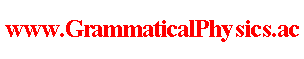since 2006 Help　Sitemap < Forum > < Problems > < Measurement Problem > < Many-worlds Solution of Uda Equation > In this page, I note down what I thought about a possibility that many-worlds interpretation is expressed and supported by my new quantum grammar. At present(2018/06/26), I have got to think that this approach is wrong. Below I use energy representation. In my new quantum grammar, Fe represents an unentangled quantum history represented by a wave-function πe in the old grammar. πe(e'', t) ＝ πe'(e'', t) if t ＜0. πe(e', t) ＝ exp[(-i/h)t]δ(e' - e) if t ＞ T. In position representation, a general wave-function ψ corresponding to a wave-function π in energy representation is as follows. ψ(x, t) ＝∫de π(e, t)ue(x) where ue is an eigenfunction of Hamiltonian with an eigenvalue e. {[-h2/(2m)](d/dx)2 + V(x)}ue(x) ＝eue(x). As for πe, position representation wave function ψe corresponding to it is as follows. ψe(x, t) ＝∫de' πe(e', t)ue'(x). I wondered if each Fe is not a solution of the Uda equation and F = ΣeCeFe is a solution of the Uda equation for some complex coefficients Ce. This idea was presented at JPS 2018 Spring Meeting and is faced with deadlock in this page. F[E] ＝ Σe Ce Fe[E]. As a sketch, Fe[E] ～ exp{α∫-∞0dt[(-i/h)tE(t)+g(E(t))+h(t)]}F'e[E(0～T)]Πτ＞Tδ(E(τ) - e). The first factor of the right hand side of this equation was derived in Energy Representation of Uda Equation @ Quantum History Theory. Precise expression may be the following one. Fe[E] ＝ δ(limt→-∞[tE(t) + ihg(E(t))])exp{α∫-∞0dt[(-i/h)tE(t)+g(E(t))+h(t)]}F'e[E(0～T)]∫DW exp{iα∫T∞dt W(t)[E(t) -e ]}. ∫-∞0dt [dE(t)/dt]δFe[E]/δE(t) 　＝ α∫-∞0dt [dE(t)/dt][(-i/h)t + ∂g(E(t))/∂E(t)] Fe[E] 　＝ α{[(-i/h)tE(t)]t=-∞0 + (i/h)∫-∞0dt E(t) + [g(E(t))]t=-∞0} Fe[E]. ∴ (-ih/α)∫-∞0dt [dE(t)/dt]δFe[E]/δE(t) 　　＝ [∫-∞0dt E(t)]Fe[E] + {[-tE(t)]t=-∞0 - ih[g(E(t))]t=-∞0}Fe[E] ∫T∞dt [dE(t)/dt]δFe[E]/δE(t) 　＝ exp{α∫-∞0dτ[(-i/h)τE(τ)+g(E(τ))+h(τ)]}F'e[E(0～T)]∫T∞dt [dE(t)/dt][δ/δE(t)]∫DW exp{iα∫T∞dτ' W(τ')[E(τ') -e ]} 　＝ 0 ∵ [E(t') - e][δ/δE(t)]∫DW exp{iα∫T∞dτ W(τ)[E(τ) - e]} 　　～ [E(t') - e]δ'(E(t) - e)Πτ≠tδ(E(τ) - e) 　　～ -δ(t' - t)δ(E(t) - e)Πτ≠tδ(E(τ) - e) 　　＝ -δ(t' - t)Πτδ(E(τ) - e) 　　＝ -δ(t' - t)∫DW exp{iα∫T∞dτ W(τ)[E(τ) - e]} 　　∴ (d/dt')[E(t') - e][δ/δE(t)]∫DW exp{iα∫T∞dτ W(τ)[E(τ) - e]} 　　　　～ -δ'(t' - t)∫DW exp{iα∫T∞dτ W(τ)[E(τ) - e]} 　　∴ [dE(t')/dt'][δ/δE(t)]∫DW exp{iα∫T∞dτ W(τ)[E(τ) - e]} 　　　　～ -δ'(t' - t)∫DW exp{iα∫T∞dτ W(τ)[E(τ) - e]} 　　∴ [dE(t)/dt][δ/δE(t)]∫DW exp{iα∫T∞dτ W(τ)[E(τ) - e]} ＝ 0. ∫T∞dt E(t) Fe[E] ＝ ∫T∞dt e Fe[E] ・・・ ※. (-ih/α)∫-∞0dt [dE(t)/dt]δF[E]/δE(t) ＝ ∫-∞0dt E(t) F[E] + {[-tE(t)]t=-∞0 - ih[g(E(t))]t=-∞0}F[E]. (-ih/α)∫T∞dt [dE(t)/dt]δF[E]/δE(t) ＝ 0. Because of these two equations, energy representation of Uda equation reduces to the following equation. (-ih/α)∫0Tdt [dE(t)/dt]δF[E]/δE(t) ＝ ∫0∞dt E(t) F[E] - {[-tE(t)]t=-∞0 - ih[g(E(t))]t=-∞0}F[E]. Because of this equation and ※, (-ih/α)∫0Tdt [dE(t)/dt]δF[E]/δE(t) ＝ ∫0Tdt E(t) F[E] + ΣeCe∫T∞dt e Fe[E] - {[-tE(t)]t=-∞0 - ih[g(E(t))]t=-∞0}F[E] ∴ ΣeCe∫0Tdt {(-ih/α)[dE(t)/dt]δ/δE(t) - E(t)}F'e[E(0～T)]∫DW exp{iα∫T∞dτ W(τ)[E(τ) - e]} 　　　＝ ΣeCe[∫T∞dt e + ihg(E(0))]F'e[E(0～T)]∫DW exp{iα∫T∞dτ W(τ)[E(τ) - e]}. I can not go further from here. I assumed that tE(t) + ihg(E(t)) → 0 as t → -∞. Below I investigated how functional differentiation is by using discretized sketch. ・・・・(a-2 + ε-2)(a-1 + ε-1)(a0 + Δ)(a1 + ε1)(a2 + ε2)・・・ ＝ ・・・・ a-2 a-1 a0 a1 a2 ・・・ + ・・・・ a-2 a-1 Δ a1 a2 ・・・ 　　+ Σk≠0(εk/ak)・・・・ a-2 a-1(a0 + Δ)a1 a2 ・・・ ∴ ・・・・(a-2 + ε-2)(a-1 + ε-1)(a0 + Δ)(a1 + ε1)(a2 + ε2)・・・ - ・・・・ a-2 a-1 a0 a1 a2 ・・・ 　　＝ ・・・・ a-2 a-1 Δ a1 a2 ・・・ + Σk≠0 εk(∂/∂ak)・・・・ a-2 a-1(a0 + Δ)a1 a2 ・・・ Fe[E] ＝ Πk=-∞0f(E(k/α), k/α)Πk=1∞δ(E(k/α) - e) Fe[E(□ - 1/α)] ＝ Πk=-∞0f(E(k/α - 1/α), k/α)Πk=1∞δ(E(k/α - 1/α) - e) 　　　　　　　　　　　＝ Πk'=-∞-1f(E(k'/α), (k' + 1)/α)Πk'=0∞δ(E(k'/α) - e) 　　　　　　　　　　　＝ Πk=-∞-1f(E(k/α), (k + 1)/α)Πk=0∞δ(E(k/α) - e)Fe[E(□ - 1/α)] - Fe[E] ＝ Πk=-∞-1f(E(k/α), k/α)[δ(E(0/α) - e) - f(E(0/α), 0/α)]Πk=1∞δ(E(k/α) - e) 　　+ Σk=-∞-1 (1/α)[∂/∂(k/α)]Πk'=-∞-1f(E(k'/α), k'/α)δ(E(0/α) - e)Πk'=1∞δ(E(k'/α) - e) I can not go further from here. I tried to calculate the shift of wave-function at t = 0. ∫DW exp{iα∫0εdτ W(τ)[E(τ) - e]} - exp{α∫0εdt[(-i/h)tE(t)+g(E(t))+h(t)]} ＝ δ(αε [E(0) - e]} - exp{αε[g(E(0))+h(0)]} if ε → +0. This also does not give any hint to me. Below I looked for Hamiltonian which causes wave contraction in measurement along the old grammar. U(t1, t2) ＝ exp[(-i/h)t1H][θ(t1)|e>